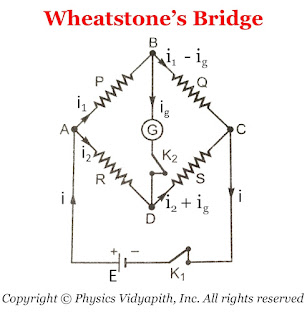### Wheatstone's Bridge

It is an arrangement of four resistance used to determine one of this resistance quickly and accurately in terms of the remaining three resistance.

Objective: To find the unknown resistance with the help of the remaining three resistance.

Principle of Wheatstone Bridge: The principle of Wheatstone bridge is based on the principle of Kirchhoff's Law.

Construction: A Wheatstone bridge consists of four resistance $P$,$Q$,$R$, and $S$. This resistance is connected to form quadrilateral $ABCD$. A battery of EMF $E$ is connected between point $A$ and $C$ and a sensitive galvanometer is connected between point $B$ and $D$ Which is shown in the figure below.Diagram of Wheatstone's Bridge

Working: To find the unknown resistance $S$, The resistance $R$ is to be adjusted like there is no deflection in the galvanometer. which means that there is not any flow of current in the arm $BD$. This condition is called "Balanced Wheatstone bridge" i.e

 $\frac{P}{Q}=\frac{R}{S}$

Derivation of Balanced Condition of Wheatstone's Bridge: In accordance with Kirchhoff's first law, the currents through various branches are shown in the figure above.

For Close Loop $ABDA$

$0=P \: I_{1} - R \: I_{2} +G \: I_{g} \qquad(1)$

For Close Loop $CBDC$

$0=Q \left( I_{1} - I_{g} \right) -S \left( I_{2} + I_{g} \right) - G\: I_{g} \qquad(2)$

For Balanced Wheatstone Bridge: $I_{g}=0$

SO from equation $(1)$ and equation $(2)$

$0=P \: I_{1} - R \: I_{2}$

$P \: I_{1} = R \: I_{2} \qquad(3)$

$0=Q I_{1} -S I_{2}$

$Q I_{1} = S I_{2} \qquad(4)$

Now divide the equation $(4)$ and equation $(3)$, then we get

 $\frac{P}{Q}=\frac{R}{S}$